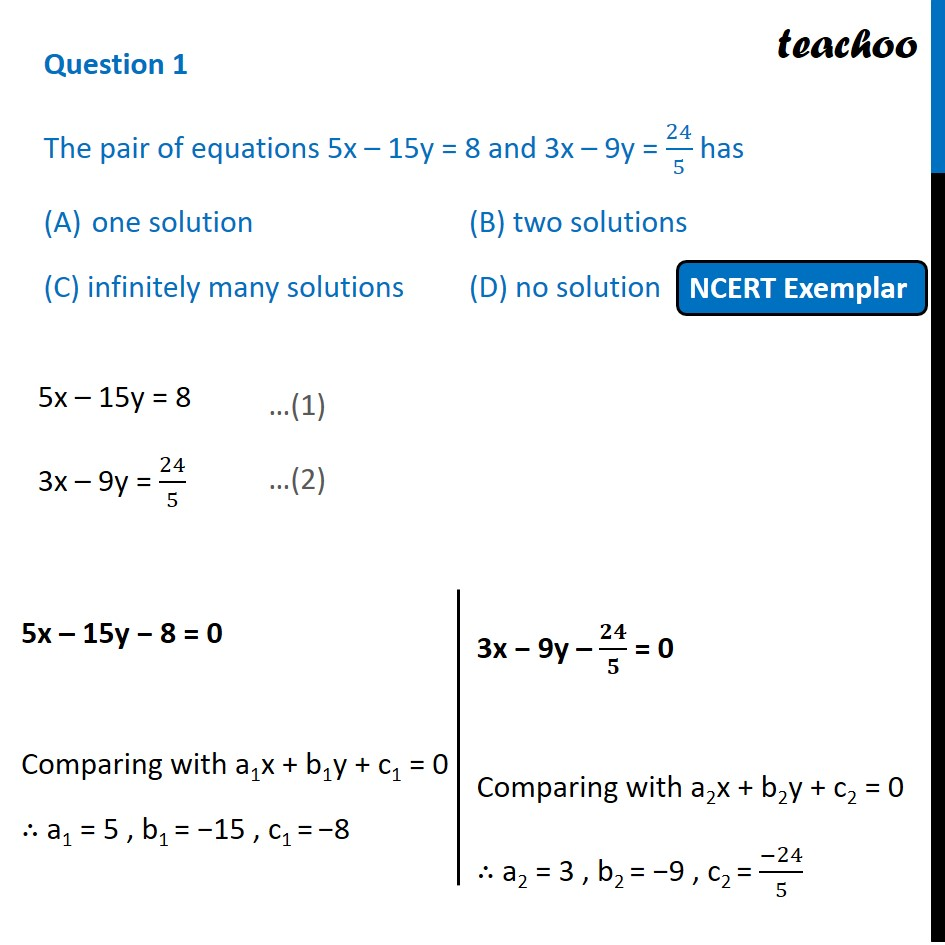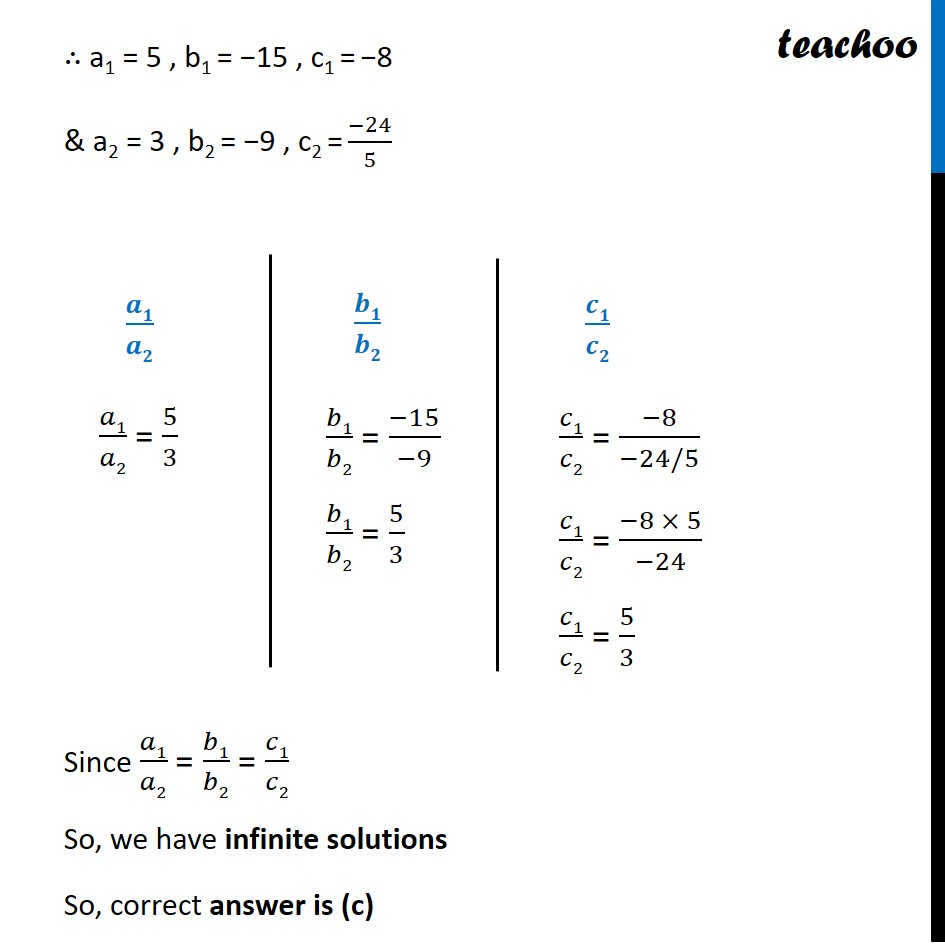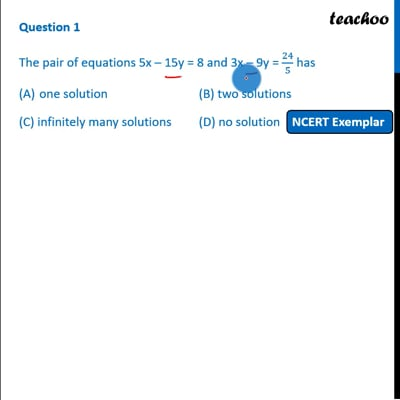MCQs from NCERT Exemplar

Chapter 3 Class 10 Pair of Linear Equations in Two Variables
Serial order wise

## (C) infinitely many solutions             (D) no solutionThis video is only available for Teachoo black users

Introducing your new favourite teacher - Teachoo Black, at only ₹83 per month

### Transcript

Question 1 The pair of equations 5x – 15y = 8 and 3x – 9y = 24/5 has one solution (B) two solutions (C) infinitely many solutions (D) no solution 5x – 15y = 8 3x – 9y = 24/5 5x – 15y − 8 = 0 Comparing with a1x + b1y + c1 = 0 ∴ a1 = 5 , b1 = −15 , c1 = −8 3x − 9y – 𝟐𝟒/𝟓 = 0 Comparing with a2x + b2y + c2 = 0 ∴ a2 = 3 , b2 = −9 , c2 = (−24)/5 ∴ a1 = 5 , b1 = −15 , c1 = −8 & a2 = 3 , b2 = −9 , c2 = (−24)/5 Since 𝑎1/𝑎2 = 𝑏1/𝑏2 = 𝑐1/𝑐2 So, we have infinite solutions So, correct answer is (c)